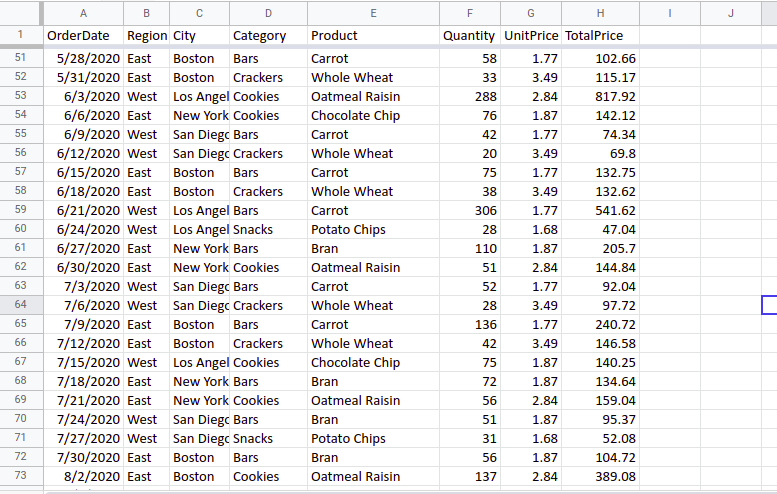Open in App
Not now

# Find the Frequency of a Particular Word in a Cell in an Excel Table in Python

• Last Updated : 03 Jan, 2023

In this article, we’ll look at how to use Python to find the number of times a word appears in a cell of an Excel file. Before we begin with the steps of the solution, the following modules/libraries must be installed. We will use the following sample Excel file to determine the frequency of the input words.

### Dataset Used

It can be downloaded from here. In this original sample table, we have a total of (99×8) Entries. Our task is to determine how many times a specific word appeared in our Excel sheet.Assume we have an excel file named sampleExcel.xlsx that contains some random data. In a cell of an Excel file, we will return the frequency of a specific word.

For example, you have an excel sheet that contains the responses of students for their academic performance and you want to count how many times a specific student entered the details by counting his/her name in the table. Exactly the same as we are doing here, counting each occurrence of a specified number or string.

### Stepwise Implementation

Step 1: In the first step, we have to import the modules, which are necessary to complete this task. The following lines of code will import the module.

Note:  We are not importing the openpyxl module because it is not required, but you must install it otherwise pandas will not work. ( In the case of Excel Sheet Manipulation ).

## Python3

 `import` `pandas as pd``from` `operator ``import` `*`

Step 2: In the second step, we take the input for the word and use typecasting to convert it to a string.

## Python3

 `# Taking input for the word``word ``=` `str``(``input``(``"Enter any word: "``))`

Step 3: The following line of code loads the Excel sheet into the pandas Dataframe. read_excel() function accepts the path of the excel file as a parameter.

## Python

 `# Loading the Excel sheet into pandas DataFrame``Xcel_file ``=` `pd.read_excel(``'FrequencyExcel\sampledatafoodsales.xlsx'``)`

Step 4: Additionally, Because the Dataframe_name[‘column_name’].value counts() function returns the frequencies of each unique value in a specific column, you can use it to manually check the frequencies for each unique word.

## Python

 `a ``=` `Xcel_file[``'Category'``].value_counts()``print``(a)`

Output:

As you can see, the <category> column is passed, and the output contains each unique word with frequencies in that column.

```Bars        39
Crackers    15
Snacks      12```

Step 5:  Suppose we take column <Category> and our word is <Cookies>. This function will return 3 ( according to the above table entries). In this step, we’ll create a frequency count and set it to zero.

`freq = 0`

Step 6: Before we go to the next lines of code we will understand a basic function and the function is countOf(pandas_dataframe_name[‘Column_name’], word).  As a parameter, we will pass the column and the input word, and the function will return the frequency of that word in that column.

```# frequency in ith column
c = countOf(Xcel_file[i], word)```

Step 7: Here, we are actually traversing each column and passing the names of each column to the previously discussed function, which will tell us the frequency of a specific word in the ith column, and we will add the frequencies of each column to get the final frequency.

## Python3

 `# Traversing each column``for` `i ``in` `Xcel_file:``    ``print``(i, ``'\n'``)` `    ``# frequency in ith column``    ``c ``=` `countOf(Xcel_file[i], word)` `    ``# incrementing the final frequency with ith column frequency``    ``freq ``+``=` `c` `# printing the final frequency in Excel table``print``(freq)`

### Complete Code:

As previously stated, the countOf() function returns the frequency of a specific word in the specified column. So we pass the same word to this function for each column name and increment the final frequency with each frequency in the ith column’s frequency.

## Python3

 `# Importing the modules``import` `pandas as pd``from` `operator ``import` `*` `# Taking input for the word``word ``=` `str``(``input``(``"Enter any word : "``))` `# Loading the Excel sheet into pandas DataFrame``Xcel_file ``=` `pd.read_excel(``'sampledatafoodsales.xlsx'``)` `# print(Xcel_file) (Optional)` `# a = Xcel_file['Category'].value_counts()` `# print(a)` `# frequency count``freq ``=` `0` `# freq = countOf(Xcel_file['Category'], "Bars")``# print(freq)` `# Traversing each column``for` `i ``in` `Xcel_file:``    ``# print(i, '\n')` `    ``# frequency in ith column``    ``c ``=` `countOf(Xcel_file[i], word)` `    ``# incrementing the final frequency with ith column frequency``    ``freq ``+``=` `c` `# printing the final frequency in Excel table``print``(freq)`

Output:

```Enter any word : Cookies
33```

### Calculate the frequency of the number in the Excel

We want to calculate the frequency of <91> number in the Excel sheet so we will pass this as input to the program and analyze the output. Here, we only change the input from a string to an integer(int).

## Python3

 `# Importing the modules``import` `pandas as pd``from` `operator ``import` `*` `# Taking input for the word``word ``=` `int``(``input``(``"Enter any word: "``))` `# Loading the Excel sheet into pandas DataFrame``Xcel_file ``=` `pd.read_excel(``'sampledatafoodsales.xlsx'``)` `# print(Xcel_file) (Optional)` `# a = Xcel_file['Category'].value_counts()` `# print(a)` `# frequency count``freq ``=` `0` `# freq = countOf(Xcel_file['Category'], "Bars")``# print(freq)` `# Traversing each column``for` `i ``in` `Xcel_file:``    ``# print(i, '\n')` `    ``# frequency in ith column``    ``c ``=` `countOf(Xcel_file[i], word)` `    ``# incrementing the final frequency with ith column frequency``    ``freq ``+``=` `c` `# printing the final frequency in Excel table``print``(freq)`

Output:

```Enter any word: 91
1```

My Personal Notes arrow_drop_up